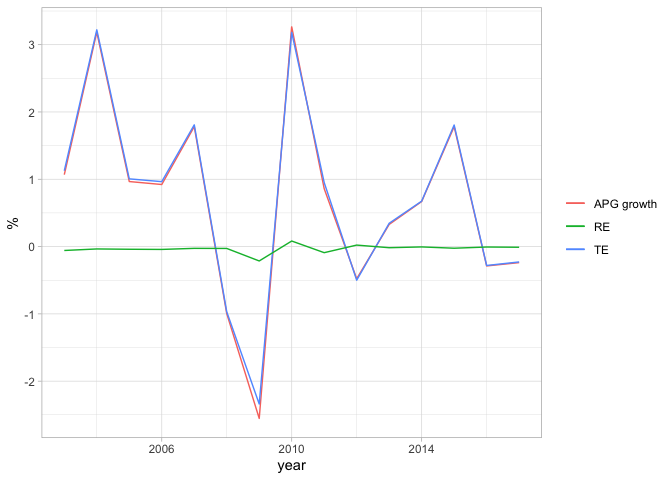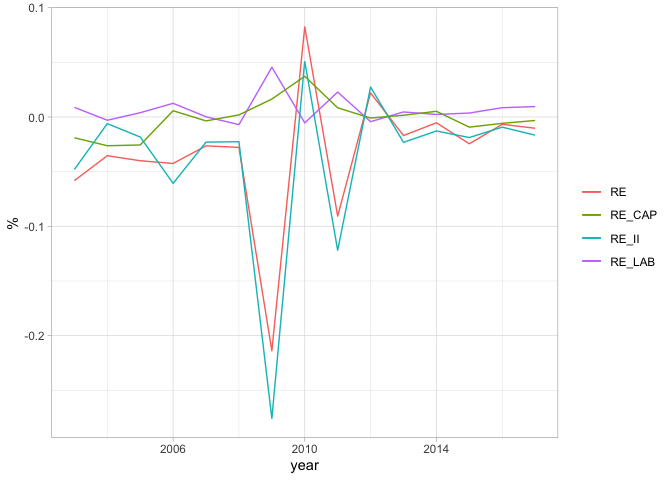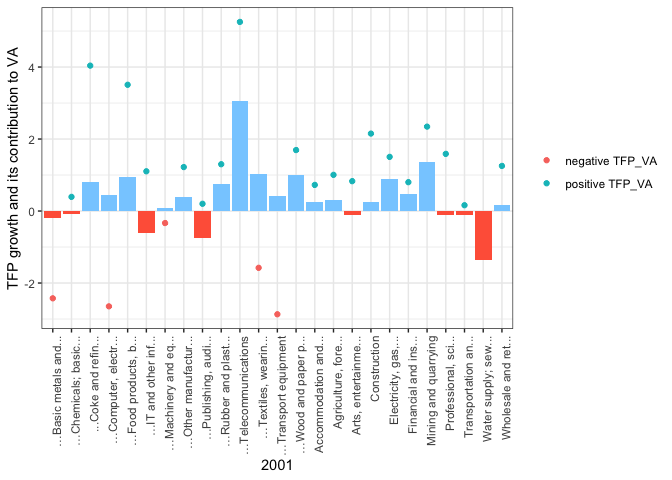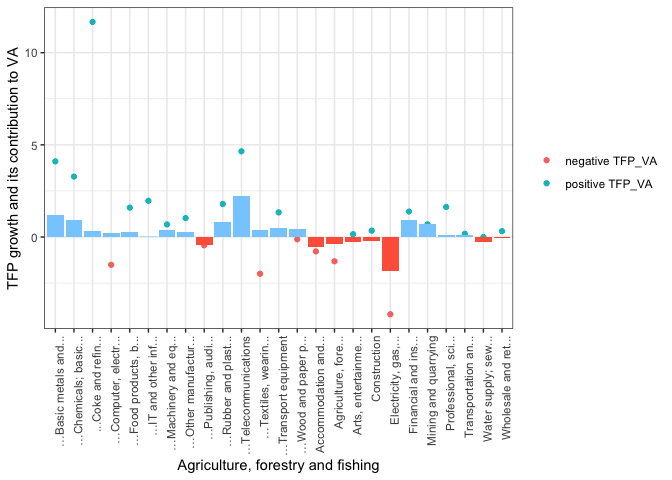# Aggregate Productivity Growth Decomposition (part 2 of 2)

Part 2 introduces the process of decomposing APG (aggregate productivity growth), taking Belgium as an example. Here, I tried to be as general and reproducible as possible. For further graphs regarding the decomposition, see here.

## Period and Demonstrative Graphs

### Data

library(knitr)
library(ggplot2)
library(dplyr)
library(plotly)
#---- changing codes here ---- #
#--change the country here
country_name <- c('Belgium')
#--change penn world data timeframe
pwt_from = 1959 #recommend from 1959 for evenly spaced
pwt_to = 2019 #recommend the latest data
pwt_interval = 10 #declare interval
#--change APG decomposition timeframe
apg_year_from = 2001 #the earliest data available, check by opening the excel file
apg_year_to = 2017 #check by opening the excel file
apg_year_interval_small = 4 #small interval for APG decomposition
apg_year_interval_big = 8 #big interval for TFP's industry decomposition
#--select variables and industries --#
variables = c("LAB","CAP","LAB_QI","CAP_QI","VA","GO","II_Q","II","VA_Q","GO_Q","EMP")
non_market_industries = c('L','O','P','Q','T','U') #edit to empty list to include
agg_industries = c("A","B","C","D","E","F","G","H","I","J","K","M_N","R_S") #grouped and aggregate industries
nagg_industries = c("A","B", "C10-C12","C13-C15","C16-C18","C19","C20_C21","C22_C23","C24_C25","C26_C27","C28","C29_C30","C31-C33","D","E","F","G","H","I","J58-J60","J61","J62_J63","K","M_N","R_S") #uncombined non aggregate industries
#for Solow:
#for APG


The development of the Belgian economy can be divided into the following sub-periods:

• 1960 - 1980: Growth was fostered by foreign investment, notably from the US
• 1980 - 1990: Following the Oil Shock in 1979 was a recession in 80-82. This period also marks the shift in the regional economic structure where Flanders gradually took Wallonia’s place as the economic center.
• 1990 - 1999: Economy headed towards more export-centric and further European integration.
• 1999 - 2009: The economy was fostered by regional dynamics and ICT’s advancement.
• 2009 - 2019: The economy is in recovering phase after the GFC.

We use EUKLEMS data here (available from 1995) Notice that both NA (national account) and GA (growth account) are necessary. Then create a subset data for Belgium.

#---- Load data ---- #
euklems_NA <- subset(dataNA, country == country_name_apg)
euklems_GA <- subset(dataGA, country == country_name_apg)


### The Idea:

1. We import important variables from the subset.
2. Calculate the Domar weights (VA and GO). From here, we can calculate $\beta$
3. $\beta$ Estimation
• Method 1: Discrete time approximation of RE term
$\int_t^{T} g_{APG}(RE) \approx \sum_i\sum_k \bar{D_{it}} (\bar{\beta}_{ik,t} - \bar{s_{ik,t}})\ d\ln(L_{ik,t}) + \sum_i\sum_j\bar{D_{it}} (\bar{\beta}_{ij,t} - \bar{s_{ij,t}})\ d\ln(X_{ij,t})$

where: $\bar{x_t} \equiv \frac{x_t + x_T}{2}$ the moving average between 2 periods

$\bar{\beta} = \frac{\sum_{t=1}^T s_t}{T}$ : elasticity as the average sum from the beginning of time until point T in time

• Method 2: Assume a functional form for productivity and estimate

For example, assume a Cobb-Douglas PF:

$Q_i = \omega L^{\beta_1}K^{\beta_2}X^{\beta_3}$

take logs and perform OLS estimation:

$\ln Q_i = \hat{\beta_0} + \hat{\beta_1} \ln(L) + \hat{\beta_2} \ln(K) + \hat{\beta_3} \ln(X) + \ln(\omega)$

PF is not necessarily CRS ($\sum \beta \ \text{can} \ne 1$).

Since it’s hard to control for the endogeneity in OLS estimation of $\beta$, we use method 1. Method can be useful for estimating TFP for each industry.

1. Divide the time periods then calculate the difference & weighted moving average
2. Perform growth accounting

### Code (hidden)

The flow:

#### Aggregate Industries

1. Subset the data

2. Calculate the Domar weights and logs of variables

3. Calculate beta

#### Aggregate industry APG | PERIODS

1. Setup the time periods and calculate dx/dt

2. Peform APG decomposition

Results:

year          g_APG_annual   TE_annual   RE_annual   RE_LAB_annual   RE_CAP_annual   RE_II_annual
2001-2005           1.6861      1.6985     -0.0130          0.0022          0.0020        -0.0172
2005-2009          -0.2286     -0.1944     -0.0341          0.0092          0.0110        -0.0543
2009-2013           0.9354      0.8894      0.0473         -0.0031          0.0160         0.0344
2013-2017           0.4626      0.4596      0.0030         -0.0010         -0.0002         0.0042


Comment:

Growth was high during the pre-GFC era.

GFC hit Belgium pretty hard but Belgium recovered fast as well.

#### Aggregate industry APG | YOY

1. Calculate the changes

2. Perform APG decomposition

Results

year   g_APG_annual   TE_annual   RE_annual   RE_LAB_annual   RE_CAP_annual   RE_II_annual
2003         1.0684      1.1266     -0.0582          0.0088         -0.0190        -0.0480
2004         3.1851      3.2205     -0.0354         -0.0030         -0.0263        -0.0061
2005         0.9661      1.0061     -0.0400          0.0039         -0.0256        -0.0183
2006         0.9220      0.9645     -0.0425          0.0125          0.0057        -0.0607
2007         1.7819      1.8083     -0.0264          0.0002         -0.0036        -0.0230
2008        -0.9968     -0.9690     -0.0278         -0.0070          0.0019        -0.0226
2009        -2.5537     -2.3398     -0.2138          0.0455          0.0164        -0.2757
2010         3.2641      3.1817      0.0824         -0.0054          0.0372         0.0506
2011         0.8595      0.9502     -0.0907          0.0228          0.0084        -0.1219
2012        -0.4780     -0.5000      0.0220         -0.0043         -0.0010         0.0274
2013         0.3290      0.3460     -0.0170          0.0046          0.0016        -0.0232
2014         0.6695      0.6748     -0.0053          0.0023          0.0052        -0.0128
2015         1.7818      1.8062     -0.0245          0.0036         -0.0093        -0.0188
2016        -0.2878     -0.2811     -0.0067          0.0085         -0.0058        -0.0093
2017        -0.2386     -0.2283     -0.0103          0.0095         -0.0032        -0.0166


The plot of APG growth, TE and RE:RE is indeed stable, meaning the economy seems to be in the frictionless state/ efficient throughout most of the time.

Gains from reallocation seem to be more pronounced following negative productivity shocks. In this case, RE was positive after the GFC, and mostly from the capital and intermediate inputs. Belgium’s economy performed pretty well after the GFC despite the European Debt Crisis during (2008-2012).

However, as the ECB was pursuing QE policy (APP) very strongly from 2016 until the end of 2018 to avoid devaluation of the Euro, the price of the Euro has steadily increased. As the result, [Belgium’s export was modest] during from 2015 to 2017 and lower than the previous years, which may have contributed to significantly slower growth during this period.

The plot of RE terms only:The movement of in labor is always positive, while CAP and II are more disruptive.

Most of the movements in RE are the result of II. Perhaps, as manufacturing and export of finished products are what Belgium is good for. Negative shift mainly due to international disruptions in the supply chain. The swift positive reallocation of II shows that domestic II is quite strong, but maybe not as competitive as imported.

#### Industry’s TFP Growth vs contribution to VA growth

Period 1:Period 2:Decling industries: computer parts, textiles, transportation, publishing, wood and paper, energy.

Raising industries: Professional, Chemicals

The share of employment in manufacturing has been declining, albeit its GO Domar weight remains roughly unchanged. A larger part of the job pool is now reserved for the Professional and scientific sector, indicating a larger role of knowledge in economic growth. The share of Domar weight of this sector to Gross Output (GO) has been also increasing over time.

Written on June 7, 2021